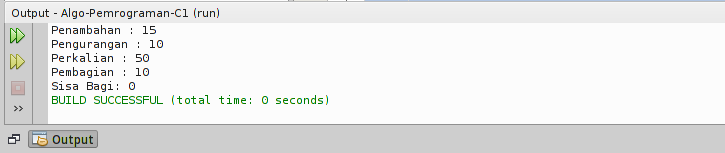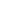| lesson material | material summary | questions and answers | definitions | types and examples | other information | materi pelajaran | ringkasan materi | pertanyaan dan jawaban | definisi | jenis-jenis dan contoh-contoh | informasi lainnya |

# Learning Java: 6 Types of Operators to UnderstandOperators in programming are used to perform certain operations.
Suppose we want to add the value of a variable `x`and `y`, then we can use the addition operator ( `+`).
``````x + y
``````
There are six types of operator groups in Java programming:
1. Artistic operators;
2. Assignment Operator;
3. Comparative Operator;
4. Logic Operator;
5. Bitwise operator;
6. and Ternary Operators.
When depicted in the mind map, it will look like this:You can see the full size of the mind map at: coggle.it
What are the differences of all types of operators?
Let's discuss them one by one…

## 1. Arithmetic Operators

Arithmetic operators are used to carry out arithmetic operations.
This operator consists of:
NameSymbol
Addition`+`
Reduction`-`
Multiplication`*`
Division`/`
Remaining Share`%`
How do you use it?

### Let's try practice ...

Create a new class called OperatorArmatics , then follow the following code:
``````import java.util.Scanner;

public class OperatorAritmatika {

public static void main(String[] args) {
int angka1;
int angka2;
int hasil;

Scanner keyboard = new Scanner(System.in);

System.out.print("Input angka-1: ");
angka1 = keyboard.nextInt();
System.out.print("Input angka-2: ");
angka2 = keyboard.nextInt();

// penjumlahan
hasil = angka1 + angka2;
System.out.println("Hasil = " + hasil);

System.out.print("Input angka-1: ");
angka1 = keyboard.nextInt();
System.out.print("Input angka-2: ");
angka2 = keyboard.nextInt();

// pengurangan
hasil = angka1 - angka2;
System.out.println("Hasil = " + hasil);

System.out.print("Input angka-1: ");
angka1 = keyboard.nextInt();
System.out.print("Input angka-2: ");
angka2 = keyboard.nextInt();

// perkalian
hasil = angka1 * angka2;
System.out.println("Hasil = " + hasil);

System.out.print("Input angka-1: ");
angka1 = keyboard.nextInt();
System.out.print("Input angka-2: ");
angka2 = keyboard.nextInt();

// Pembagian
hasil = angka1 / angka2;
System.out.println("Hasil = " + hasil);

System.out.print("Input angka-1: ");
angka1 = keyboard.nextInt();
System.out.print("Input angka-2: ");
angka2 = keyboard.nextInt();

// Sisa Bagi
hasil = angka1 % angka2;
System.out.println("Hasil = " + hasil);

}

}
``````Easy isn't it ...?

### Want laithan again?

The more practice, the more steady.
Now try the case example: The program calculates the circumference of a rectangle.## 2. Assignment operator

The assignment operator (Operator Assignment) function to gave the duty on certain variables. Usually to fill in the value.
Example:
``````int a = 10;
``````
Variables are `a`assigned to store values `10`.
Assignment operator consists of:
Operator NameSombol
Charging Value`=`
Filling and Addition`+=`
Charging and Reduction`-=`
Filling and Multiplication`*=`
Filling and Distribution`/=`
Charging and Remaining for`%=`

### Let's Try ...

Now, make a new class called Operator Assignment .
``````public class OperatorPenugasan {

public static void main(String[] args) {
int a;
int b;

// Pengisian nilai
a = 5;
b = 10;

// penambahan
b += a;
// sekarang b = 15
System.out.println("Penambahan : " + b);

// pengurangan
b -= a;
// sekarang b = 10 (karena 15-5)
System.out.println("Pengurangan : " + b);

// perkalian
b *= a;
// sekarang b = 50 (karena 10*5)
System.out.println("Perkalian : " + b);

// Pembagian
b /= a;
// sekarang b=10
System.out.println("Pembagian : " + b);

// Sisa bagi
b %= a;
// sekarang b=0
System.out.println("Sisa Bagi: " + b);

}

}
``````
The output results:## 3. Pambanding operator

As the name implies, the task of the opera is to compare.
This operator is also known as an opeartor relation.
The value generated from this operator is boolean, namely: `true` and `false`.
This operator consists of:
NameSymbol
Greater than`>`
Smaller`<`
Together with`==`
Not equal to`!=`
Bigger Same with`>=`
Smaller Same as`<=`
example:
``````boolean x = 10 < 12
``````
Then it `x`will be true , because it `10`is smaller than `12`.
For more details…

### Let's try in code

Make a new class called Operator Comparator . Then follow the following code:
``````public class OperatorPembanding {

public static void main(String[] args) {
int nilaiA = 12;
int nilaiB = 4;
boolean hasil;

// apakah A lebih besar dari B?
hasil = nilaiA > nilaiB;
System.out.println(hasil);

// apakah A lebih kecil dari B?
hasil = nilaiA < nilaiB;
System.out.println(hasil);

// apakah A lebih besar samadengan B?
hasil = nilaiA >= nilaiB;
System.out.println(hasil);

// apakah A lebih kecil samadengan B?
hasil = nilaiA <= nilaiB;
System.out.println(hasil);

// apakah nilai A sama dengan B?
hasil = nilaiA == nilaiB;
System.out.println(hasil);

// apakah nilai A tidak samadengan B?
hasil = nilaiA != nilaiB;
System.out.println(hasil);

}

}
``````
The program code above will produce an output like this:## 4. Logic Operators

If you have studied mathematical logic, you will definitely not be familiar with this operator.
NameSymbol in Java
Logic AND`&&`
Logic OR`||`
Negation / reverse`!`
Logic operators are used to make logical operations.
For example like this:
• Statement 1: Farmer Code a programmer
• Harvest 2: Code using Linux
If asked, is it a Farmer Code programmer who uses Linux?
Of course we will check the truth first
• Statement 1: Farmers Code a programmer = `true`.
• Harvest 2: Code using Linux = `true`.
What are programmers and programmers using Linux?
``````Pertnyataan 1 && Pernyataan 2 = true
``````
Confused?
Try checking the truth table for logic AND.
Statement 1Statement 2Logic AND
`true``true``true`
`true``false``false`
`false``true``false`
`false``false``false`
Still confused…?
It seems that you have to open up the subject of mathematical logic.
Then how do you use it in the program?

### Immediately try the following program immediately

Make a new class called OperatorLogika . Then follow the following code:Please run and pay attention to the results:## 5. Bitwise operator

The bitwise operator is an operator that is used for bit (binary) operations. The bitwise operator consists of:
NameSymbol in Java
AND`&`
OR`|`
XOR`^`
Negation / reverse`~`
Left Shift`<<`
Right Shift`>>`
Left Shift (unsigned)`<<<`
Right Shift (unsigned)`>>>`
This operator applies for the data type `int``long``short``char`, and `byte`.
This operator will calculate from bit-to-bit.
For example, we have variables `a = 60`and `b = 13`.
When made in binary form, it will be like this:
``````a = 00111100
b = 00001101
``````
Then, do the bitwise operation
Operation AND
``````a = 00111100
b = 00001101
a & b = 00001100
``````
OR operation
``````a = 00111100
b = 00001101
a | b = 00111101
``````
XOR operation
``````a = 00111100
b = 00001101
a ^ b = 00110001
``````
NOT Opportunity
``````a = 00111100
~a  = 11000011
``````
The concept is almost the same as Opeartor Logic. The difference is, Bitwise is used for binary.
For more details…

### Let's try in the program

Create a new class with a name `OperatorBitwise`, then follow the contents as follows.
``````public class OperatorBitwise {

public static void main(String[] args) {
int a = 60;    /* 60 = 0011 1100 */
int b = 13;    /* 13 = 0000 1101 */
int c = 0;

c = a & b;       /* 12 = 0000 1100 */
System.out.println("a & b = " + c);

c = a | b;       /* 61 = 0011 1101 */
System.out.println("a | b = " + c);

c = a ^ b;       /* 49 = 0011 0001 */
System.out.println("a ^ b = " + c);

c = ~a;          /*-61 = 1100 0011 */
System.out.println("~a = " + c);

c = a << 2;     /* 240 = 1111 0000 */
System.out.println("a << 2 = " + c);

c = a >> 2;     /* 215 = 1111 */
System.out.println("a >> 2  = " + c);

c = a >>> 2;     /* 215 = 0000 1111 */
System.out.println("a >>> 2 = " + c);
}

}
``````
Look at the output:

## 6. Ternary operators

This operator is unique, like making questions.
The symbol uses a question mark ( `?`) and two-point ( `:`) to separate the answer.In the example above, "You like me" is the question or condition that will be examined.
If the answer is correct, then `iya`Otherwise it will `tidak`.
For more details, let's try ...

### Program with Ternary Operators

Make a new class with a name `OperatorTernary`, then follow the following code.
``````public class OperatorTernary {
public static void main(String[] args) {

boolean suka = true;
String jawaban;

// menggunakan operator ternary
jawaban = suka ? "iya" : "tidak";

// menampilkan jawaban
System.out.println(jawaban);

}
}
``````
The output results:
``````iya
``````
Now try changing the variable value `suka`to `false`, then run again.
Will definitely produce `tidak`.
Another way, can also make conditions like this:
``````int suka = 8;

String jawaban = (suka > 5) ? "iya" : "tidak";``````
`Sumber : https://www.petanikode.com/java-operator/`
##### 0 Komentar untuk "Learning Java: 6 Types of Operators to Understand"

Silahkan berkomentar sesuai artikel

Template By Kunci Dunia